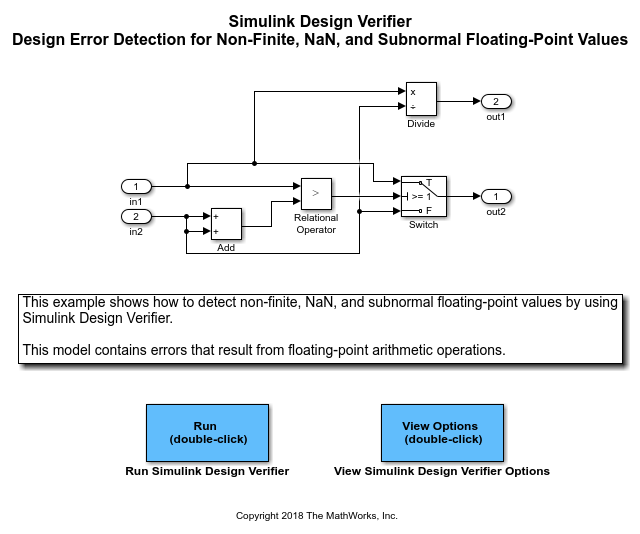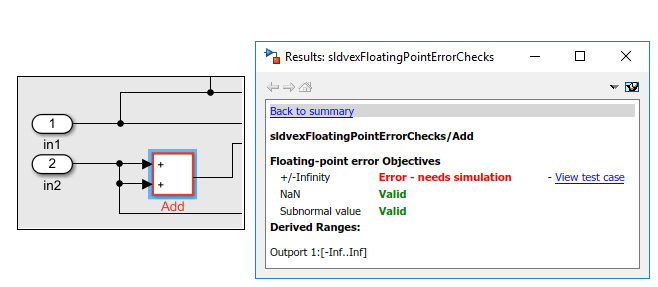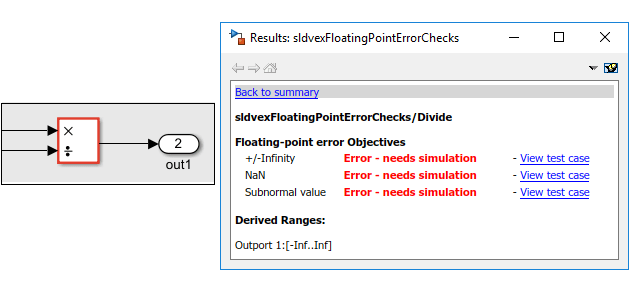Main Content

## Detect Non-Finite, NaN, and Subnormal Floating-Point Values

To detect occurrences of nonfinite, NaN, and subnormal floating-point values in a model:

1. On the Design Verifier tab, in the Mode section, select Design Error Detection.

2. Click .

3. In the Configuration Parameters dialog box, in Design Error Detection pane:

1. Select the check box for Non-finite and NaN floating-point values.

2. Select the check box for Subnormal floating-point values.

3. To apply these settings, click and close the Configuration Parameters dialog box.

4. Click .

Simulink® Design Verifier™ analyzes the model to detect the occurrences of nonfinite, NaN, and subnormal floating-point values.

After the analysis is complete:

• The software highlights the model with the analysis results.

• The Results Summary windows displays the summary of the analysis.

### Assumptions and Limitations

When you analyze a model and select Non-finite and NaN floating-point values, the software assumes that the floating-point input values and the tunable parameter values are finite.

When you analyze a model and select Subnormal floating-point values, the software assumes that the floating-point input values and the tunable parameter values are normal.

Models that use double-precision floating-point signals take more time to analyze than similar models that use single-precision floating-point signals. As a result, models that use double-precision floating-point signals might time out whereas similar models that use single-precision floating-point signals complete their analysis. To improve analysis performance, consider specifying minimum and maximum values that mimic environmental constraints on root-level Inport blocks.

If the model contains cast operations between floating-point signals and multiword fixed-point signals, the analysis might not be able to decide all objectives.

### Run Design Error Detection Analysis to Detect Floating-Point Errors

This example shows how to detect nonfinite, NaN, and subnormal floating-point values in the `sldvexFloatingPointErrorChecks` example model. The model consists of floating-point arithmetic operations that result in an error. Perform design error detection analysis to detect these errors in the model.

1. Open the Model

This example model consists of Add and Divide blocks that handle floating-point calculations. The design error detection analysis detects the occurrences of floating-point errors in the model and reports the results.

```open_system('sldvexFloatingPointErrorChecks'); ```2. Perform Design Error Detection Analysis

The model is preconfigured with Non-finite and NaN floating-point values and Subnormal floating-point values options set to On. For more information see Design Verifier Pane: Design Error Detection.

To perform design error detection analysis, on the Design Verifier tab, in the Mode section, select Design Error Detection. Click Detect Design Errors.

The software analyzes the model for floating-point errors and displays the results in the Results Summary window. The result indicates that `4` out of `6` objectives are falsified.

3. Review Analysis Results

a. Click Highlight analysis results on model. The model blocks that result in floating-point errors are highlighted in red.

b. Click the Add block highlighted in red. The Result Inspector displays the summary of the floating-point error objectives.c. Click the Division block highlighted in red. The Result Inspector displays the summary of the floating-point error objectives.4. View Detailed Analysis Report

To view the detailed analysis report, in the Results Summary window, click HTML. The report displays the summary of all occurrences of floating-point errors in the model.5. Clean Up

To complete this example, close the model.

```close_system('sldvexFloatingPointErrorChecks', 0); ```

Get resources